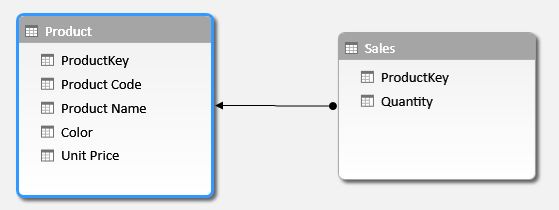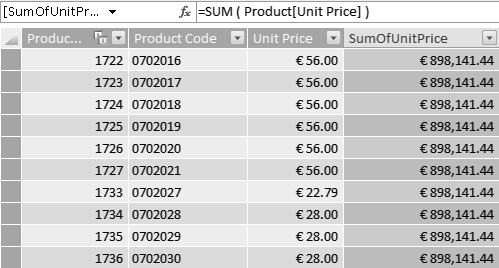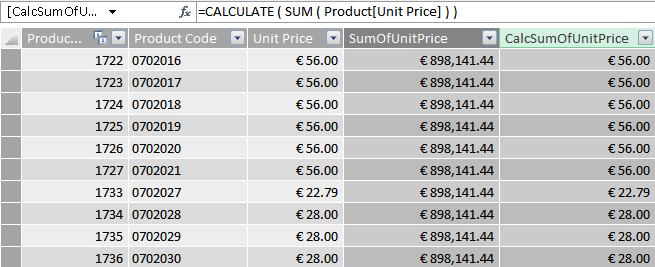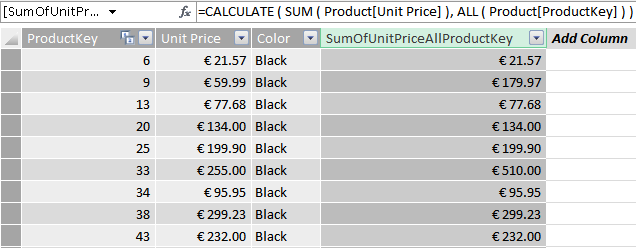Understanding context transition in DAX.

Context transition is an operation performed by CALCULATE and CALCULATETABLE in the definition of the new filter context, under which it evaluates its expression. The formal definition of context transition is easy, but it hides some complexities. In the following description you will see examples based on CALCULATE, but all the concepts can be applied to CALCULATETABLE as well.

CALCULATE transforms all existing row contexts into an equivalent filter context before applying its filter arguments to the original filter context. This happens during the creation of the new filter context to execute its expression parameter.

Context transition is easier to observe through examples than to learn by theory. For example, consider a data model containing only Product and Sales, with a relationship based on ProductKey:You can create a calculated column in Product:

```Product[SumOfUnitPrice] = SUM ( Product[Unit Price] )
```

Being a calculated column, it is computed in a row context. Nevertheless, because SUM computes the sum of all unit prices visible in the current filter context, the result is, for each product, the sum unit prices from the entire table. In a calculated column there is no filter context, only a row context.Many DAX novices erroneously think that the result of the calculated column should be the value of Unit Price in the current row only. As soon as they master row and filter context, the behavior becomes very natural. What happens if you create a column with the following code, which includes a call to CALCULATE?

```Product[CalcSumOfUnitPrice] = CALCULATE ( SUM ( Product[Unit Price] ) )
```

This time, CALCULATE surrounds SUM, so SUM is executed in a different filter context. Because CALCULATE has no filter parameter, its only effect is context transition. The row context, which contains a single row, is transformed into a filter context that contains the same row. At this point, SUM runs into a filter context with a single row and returns the value of Unit Price for that row only:You can observe the same behavior when using SUM with a column of Sales, as in the following two calculated columns:

```Product[SumOfSalesQuantity] = SUM ( Sales[Quantity] )
Product[CalcSumOfSalesQuantity] = CALCULATE ( SUM ( Sales[Quantity] ) )
```

The first calculated column returns the grand total of Sales[Quantity], because no filter context is active, whereas the one with CALCULATE returns the sum of Sales[Quantity] for the current product only, because the filter context containing the current product is automatically propagated to sales due to the relationship between the two tables:There is a big difference between the filter context introduced by context transition and the row context. In fact, the filter context generated by CALCULATE places a filter on all the columns of the table to identify a single row, not on its row number. Thus, if you use context transition in a table that contains duplicated rows, the filter context generated by CALCULATE includes all the duplicates. Thus, it is safe to rely on context transition to filter a single row if and only if the table does not contain any duplicated row. This, of course, holds true if the table has a primary key that guarantees the absence of duplication.

It is important to note that context transition happens before further filters in CALCULATE. Thus, filters in CALCULATE might override filters produced by context transition. In the next example, Product has only three columns: ProductKey, Unit Price and Color. If you define a calculated column using this code:

```Product[SumOfUnitPriceAllProductKey] =
CALCULATE (
SUM ( Product[Unit Price] ),
ALL ( Product[ProductKey] )
)
```

ALL removes any filter from ProductKey and, because it is applied after context transition, it also removes the filter on ProductKey introduced by context transition. As a result, the calculated column computes the sum of Unit Price for all the products with the same color and unit price:It is worth remembering that when you invoke a measure from a DAX expression, this is automatically surrounded by CALCULATE. The automatic CALCULATE, added by DAX, performs context transition and it might become source of errors in your code, if you do not pay attention to it.

If, for example, you want to query a model returning the products that sold more than 1% of the total sales, you might be tempted to write the query in this way:

```DEFINE
MEASURE Product[TotalSales] =
SUM ( Sales[Quantity] )
EVALUATE
FILTER (
VALUES ( Product[Product Code] ),
"SalesOfProduct", CALCULATE ( [TotalSales] )
),
[SalesOfProduct] >= [TotalSales] * 0.01
)
```

Please note the explicit CALCULATE surrounding the call to TotalSales inside ADDCOLUMNS (line 8), which suggest that context transition is required there, to compute the sales of the currently iterated product only. In reality, TotalSales is a measure, so CALCULATE is not required because DAX automatically adds it. Worse, the query does not work because the engine adds a CALCULATE around the second call to TotalSales in the condition of FILTER (line 10), where we compare SalesOfProduct with 1% of TotalSales. Since CALCULATE is there, the query really checks the sales of a product agains 1% of the sales of the same product.

In order to produce the correct result, you can write the query in this way:

```DEFINE
MEASURE Product[TotalSales] =
SUM ( Sales[Quantity] )
EVALUATE
FILTER (
VALUES ( Product[Product Code] ),
"SalesOfProduct", [TotalSales]
),
[SalesOfProduct] >= SUM ( Sales[Quantity] ) * 0.01
)
```

As you can see, we removed the useless (because implicit) CALCULATE around the first call of TotalSales (line 8). Then, we used SUM ( Sales[Quantity] ) in the condition used by FILTER (line 10), in order to avoid the implicit context transition.

The previous query can be written in a more elegant way by taking advantage of variables:

```DEFINE
MEASURE Product[TotalSales] = SUM ( Sales[Quantity] )
VAR SalesOfAllProducts = [TotalSales]
EVALUATE
FILTER (
VALUES ( Product[Product Code] ),
"SalesOfProduct", [TotalSales]
),
[SalesOfProduct] >= SalesOfAllProducts * 0.01
)
```

Variables in DAX helps you evaluating an expression in a different context other than the one where you want to use its result. You can find more examples in Variables in DAX.

CALCULATEContext transition

Evaluates an expression in a context modified by filters.

`CALCULATE ( <Expression> [, <Filter> [, <Filter> [, … ] ] ] )`

CALCULATETABLEContext transition

Evaluates a table expression in a context modified by filters.

`CALCULATETABLE ( <Table> [, <Filter> [, <Filter> [, … ] ] ] )`

SUMAdds all the numbers in a column.

`SUM ( <ColumnName> )`

ALLCALCULATE modifier

Returns all the rows in a table, or all the values in a column, ignoring any filters that might have been applied.

`ALL ( [<TableNameOrColumnName>] [, <ColumnName> [, <ColumnName> [, … ] ] ] )`

ADDCOLUMNSReturns a table with new columns specified by the DAX expressions.

`ADDCOLUMNS ( <Table>, <Name>, <Expression> [, <Name>, <Expression> [, … ] ] )`

FILTERReturns a table that has been filtered.

`FILTER ( <Table>, <FilterExpression> )`